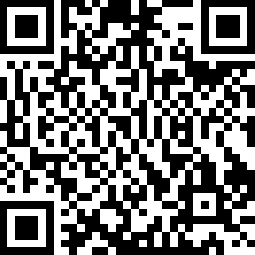﻿ SOLUTION — Second Glances

### Solution

This is a straight-up nonogram in which the solution is a QR code.The QR code leads to the Logfile Analyzer with a fake logfile in which the “logging” for each “module” contains a clue for one letter.

#HintLetter
1The number of batteries3 = C
2The starting time in minutes15 = O
3The sum of the digits in the serial number13 = M
4The number of duplicated port types, squared16 = P
5The number of strikes1 = A
6The sum of the letters in the lit indicator19 = S
7Hint #1 + Hint #419 = S
8The number of ports9 = I
9The sum of the letters in the serial number15 = O
10The last digit of the serial number times the number of battery holders14 = N

COMPASSION